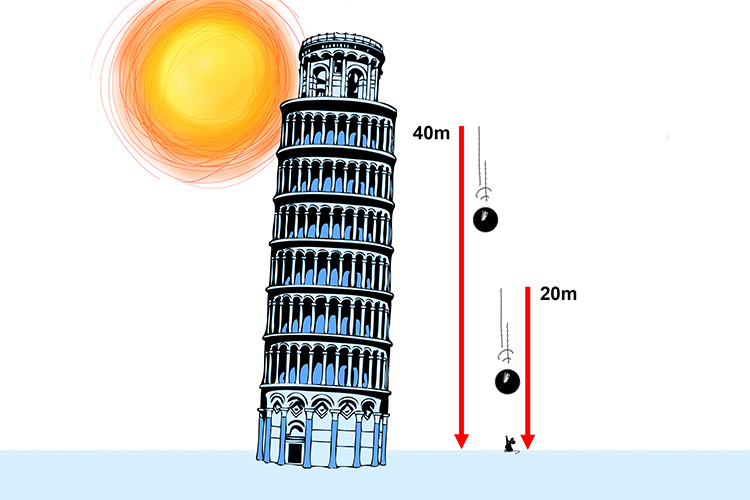# Gravitational potential energy

Gravitational potential energy is the energy possessed by an object due to its position in a gravitational field. The formula for calculating gravitational potential energy is:

Gravitational potential energy = mass x gravitational field strength x height movedGold plate even my grand house

Gravitational potential energy is measured in Joules (J). To remember this, think of the following image:Everything was gold plated but my jewels.

NOTE:

An object's gravitational potential energy is converted to other forms of energy (usually kinetic energy) as gravity pulls the object towards the centre of the gravitational field.

For example, when a ball is dropped from a tower, it falls towards the ground (towards the centre of the gravitational field). The ball's gravitational potential energy is reduced as it falls, but its kinetic energy (movement) increases.Above: when a 1kg ball is released at the top of the tower it has 0 kinetic energy but has a gravitational potential energy of GPE = 1 x 10 x 40 = 400J.

But by the time the ball has fallen to 20m above the ground, its GPE = 1 x 10 x 20 = 200J, meaning it will have gained 200J of kinetic energy by this point.

As the ball hits the ground, all of the gravitational potential energy it had at the top of the tower will have been converted to kinetic energy.

Example 1

An owl has a mass of 4kg. It dives to catch a mouse, losing 800 Joules of gravitational potential energy. Assuming acceleration due to gravity to be 10 m/s2 how high was the bird to begin with?

Gravitational potential energy = mass x gravitational field strength x height moved

800 = 4 x 10 x owl's change in height

Divide both sides by 4 x 10 to make owl's change in height the subject,

\frac{800}{4\ \times10}\ =\ \frac{\strike{4\ \times10\ }\times Owl's\ change\ in\ height}{\strike{4\ \times10}}

Owl's\ change\ in\ height\ \frac{800}{4\ \times\ 10}\ =\ \frac{800}{40}=\ 20m

Example 2

An astronaut wearing his suit has a total mass of 110kg. On the moon, he climbs into his spacecraft 5m up a ladder. This increases his GPE by 880 Joules. What is the strength of gravity on the moon?

Note

Gravity can be measured in N/kg but can also be given as m/s2 (metres per second squared). However, if the question asks for the gravitational field strength you should give your answer as N/kg and if it asks for the acceleration due to gravity then your answer should be given as m/s2.

Gravitational potential energy = mass x gravitational field strength x height moved

880 = 110 x gravity x 5

880 = 110 x 5 x gravity

Divide both sides by 110 x 5 to make gravity the subject,

\ \frac{880}{110\times5}\ =\ \frac{\strike{110\times5\ }\times gravity\ }{\strike{110\ \times5}}

gravity\ =\ \frac{880}{110\times5\ }=1.6N/kg

Example 3

 Object Mass of object Elephant 5 tonnes Person 80 Kg

Elephants don’t jump. But if they could how much more gravitational energy would one have if it jumped 30cm compared to a person who jumped the same height? Assuming acceleration due to gravity is 10m/s2.